### 15.2.1.3 Variants of the HJB equation

Several versions of the HJB equation exist. The one presented in (15.14) is suitable for planning problems such as those expressed in Chapter 14. If the cost-to-go functions are time-dependent, then the HJB equation is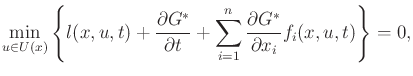(15.16)

and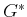is a function of bothand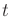. This can be derived again using a Taylor expansion, but withandtreated as the variables. Most textbooks on optimal control theory present the HJB equation in this form or in a slightly different form by pulling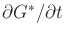outside of the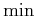and moving it to the right of the equation: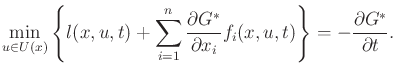(15.17)

In differential game theory, the HJB equation generalizes to the Hamilton-Jacobi-Isaacs (HJI) equations [59,477]. Suppose that the system is given as (13.203) and a zero-sum game is defined using a cost term of the form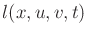. The HJI equations characterize saddle equilibria and are given as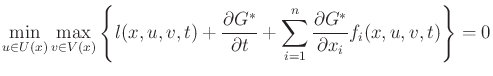(15.18)

and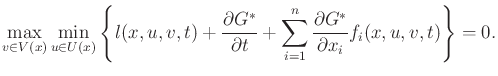(15.19)

There are clear similarities between these equations and (15.16). Also, the swapping of theand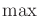operators resembles the definition of saddle points in Section 9.3.

Steven M LaValle 2020-08-14